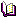[ Data Screening || Matrix Algebra with SAS/IML || Regression analysis || General Linear Models || Canonical correlation, Discriminant analysis || Logistic Regression || Factor Analysis || Clustering and scaling || SAS macro programs || Data sets || Programs]

## SAS macro programs

These macro programs are stored in an "autocall" library on the Hebb server, so you don't need to do anything special to have them included in your work. Simply include lines to call the macro program. For example (assuming you have already read in the AUTO dataset),
```  %boxcox(data=auto, resp=MPG, model=Weight Displa Gratio);
```
will search for and load the BOXCOX macro (if it has not already been loaded) and invoke the macro with the AUTO data. See the notes on The SAS Macro Facility for information on SAS macros.

For those items below which have links, selecting the name will take you to brief documentation of the macro program. The main links for these programs are:

Within SAS for Windows in the Psych Lab, online documentation may also be obtained using the WEBHELP macro. For example,
```%webhelp(boxcox);
```
brings up (in Firefox) documentation on the BOXCOX macro.
biplot.sasImplements the biplot technique (e.g., Gabriel, 1971) for plotting multivariate observations and variables together in a single display.
boxcox.sasFinds power transformations of the response variable in a regression model (PROC REG) by the Box-Cox method, with graphic display of the maximum likelihood solution, t-values for model effects, and the influence of observations on choice of power.
boxglm.sasFinds power transformations of the response variable in a general linear model (PROC GLM) by the Box-Cox method.
canplot.sasCanonical discriminant structure plot. Displays the observations in a dataset in the space of the two canonical variables which discriminate most among groups. Also plots class means on the canonical variates, confidence circles for those means, and variable vectors showing the correlations of variables with the canonical variates.
corresp.sasMacro for correspondence analysis for analysis of patterns of association in a contingency table. Similar to the CORRESP procedure, but produces a data set of point labels for plotting, an produces plots with properly equated axes.
cpplot.sasPlots of Mallow's C(p) and related statistics for model selection in linear models
cqplot.sasQQ comparison plots for multivariate normal data, with confidence envelopes.
csmpower.sasPower analysis for Covariance Structure Models
faces.sasDraws (possibly asymmetric) faces to represent multivariate data.
gtree.sasGraphic display of a clustering tree, using the result of either PROC CLUSTER or PROC VARCLUS.
inflogis.sasProduces an influence plot for a logistic regression model. The plot shows a measure of badness of fit for a given case (DIFDEV or DIFCHISQ) vs. the fitted probability (PRED) or leverage (HAT), using an influence measure (C or CBAR) as the size of a bubble.
inflplot.sasProduces an influence plot for a regression model -- a plot of studentized residuals vs. leverage (hat-value), using COOK's D or DFFITS as the size of a bubble symbol.
linpro.sasLinearly optimal profiles algorithm. For a set of multivariate observations, this algorithm (from Hartigan, 1977) finds scale positions for the variables along a single dimension so that the profile traces of the observations are as nearly linear as possible.
meanplot.sasPlot means for factorial designs: plots 1-way, 2-way, or 3-way means for a factorial design with any number of factors.
mpower.sasMultivariate retrospective power analysis Similar to the RPOWER macro, but calculates power for the multivariate tests based on Wilks' Lambda, Pillai Trace criterion, Lawley/Hotelling Trace, and Roy's Largest Root criterion.
outlier.sasMultivariate outlier detection The OUTLIER macro calculates robust Mahalanobis distances by iterative multivariate trimming (Gnanadesikan & Kettenring, 1972; Gnanadesikan, 1977), and produces a chisquare Q-Q plot.
partial.sasProduces high-resolution partial regression residual plots. Observations with high leverage and/or large studentized residuals can be individually labeled.
rpower.sasRetrospective power analysis. Takes the OUTSTAT dataset from a PROC GLM analysis and computes the power for each effect tested, as if the means were population means.
rsqdelta.sasCompute R-square change and F-statistics in regression.
scatmat.sasDraws a scatterplot matrix for all pairs of variables. A classification variable may be used to assign the plotting symbol and/or color of each point.
scatter.sasScatterplot matrix using SAS/INSIGHT.
symbox.sasBoxplots for transformations to symmetry.